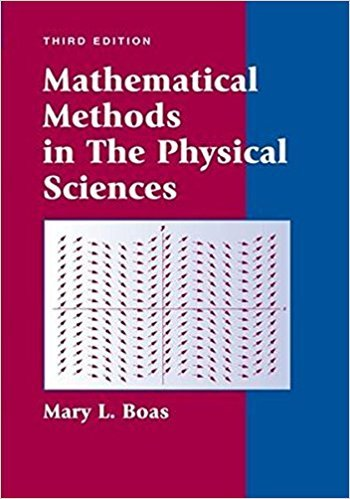### Solution Found!# Derive the formula (1.4) for the sum Sn of the geometric progression Sn = a + ar + ar2 +

Chapter 1, Problem 2

(choose chapter or problem)

Get Unlimited Answers! Check out our subscriptions
QUESTION:

Derive the formula (1.4) for the sum Sn of the geometric progression Sn = a + ar + ar2 + + arn1. Hint: Multiply Sn by r and subtract the result from Sn; then solve for Sn. Show that the geometric series (1.6) converges if and only if |r| < 1; also show that if |r| < 1, the sum is given by equation (1.8).

QUESTION:

Derive the formula (1.4) for the sum Sn of the geometric progression Sn = a + ar + ar2 + + arn1. Hint: Multiply Sn by r and subtract the result from Sn; then solve for Sn. Show that the geometric series (1.6) converges if and only if |r| < 1; also show that if |r| < 1, the sum is given by equation (1.8).

Step 1 of 2

Given(i)

To derive a formula for.

Multiplyingwith the both sides of equation (i),(ii)

Subtracting equation (ii) from equation (i),Thus, the sum of a geometric series with first termand common ratiois formulated by